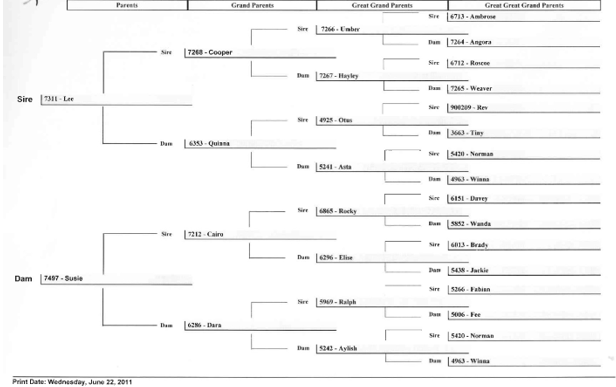Location:

# Appendix A: Calculate an Inbreeding Coefficient

The greater the number of generations of data available, the more accurate an inbreeding coefficient will be. If there is minimal data logged on the database, a coefficient can be calculated manually from a pedigree tree.

A dog will inherit half it’s alleles from the dam and half it’s alleles from the sire. The inbreeding coefficient calculates the probability that a dog has received the same allele from both dam and sire.

Take the following pedigree of KirriWinna is 3 generations away from both dam and sire of Kirri.

As each generation inherits half its alleles from each parent, then the likelihood of a same allele from Winna carrying to Suzie is ½ x ½ x ½ = ½ 3 = 0.125.

Also the likelihood that the same allele is carried from Winna to Lee is ½ x ½ x ½ = ½3 = 0.125.

We calculate the inbreeding coefficient of Kirri by multiplying the risk of getting an allele from each parent and then halving that value as Kirri is another generation away from Winna.

Therefore the inbreeding coefficient of Kirri is (½ x ½ x ½) X (½ x ½ x ½) / 2 = 1/26 / 2 = 0.0078125 = 0.78%.

This calculation assumes that Winna is not inbred. If Winna was inbred and had an inbreeding coefficient of 3%, then the probability of Suzie and Lee inheriting that allele is 3% greater, therefore the calculation becomes (½ 6 x 1.03) / 2 = 0.00804 = 0.80%.

If there was more than one common ancestor, then the calculations are made for each ancestor and added together to determine the inbreeding coefficient